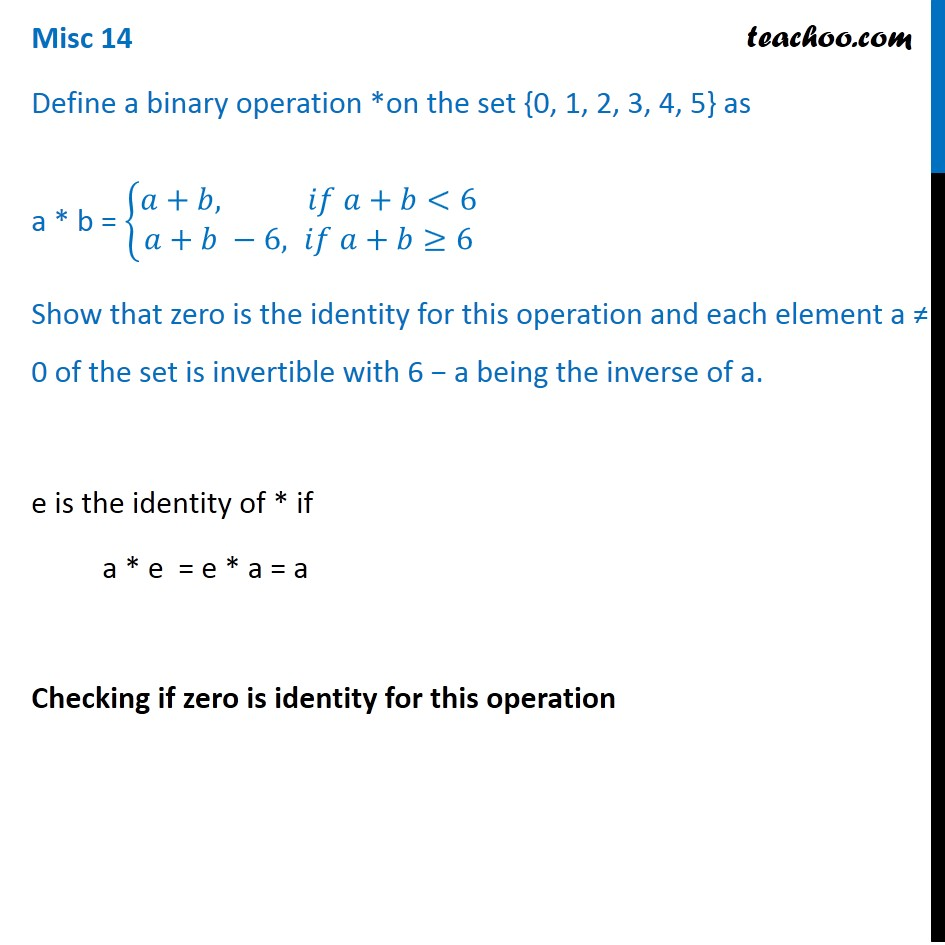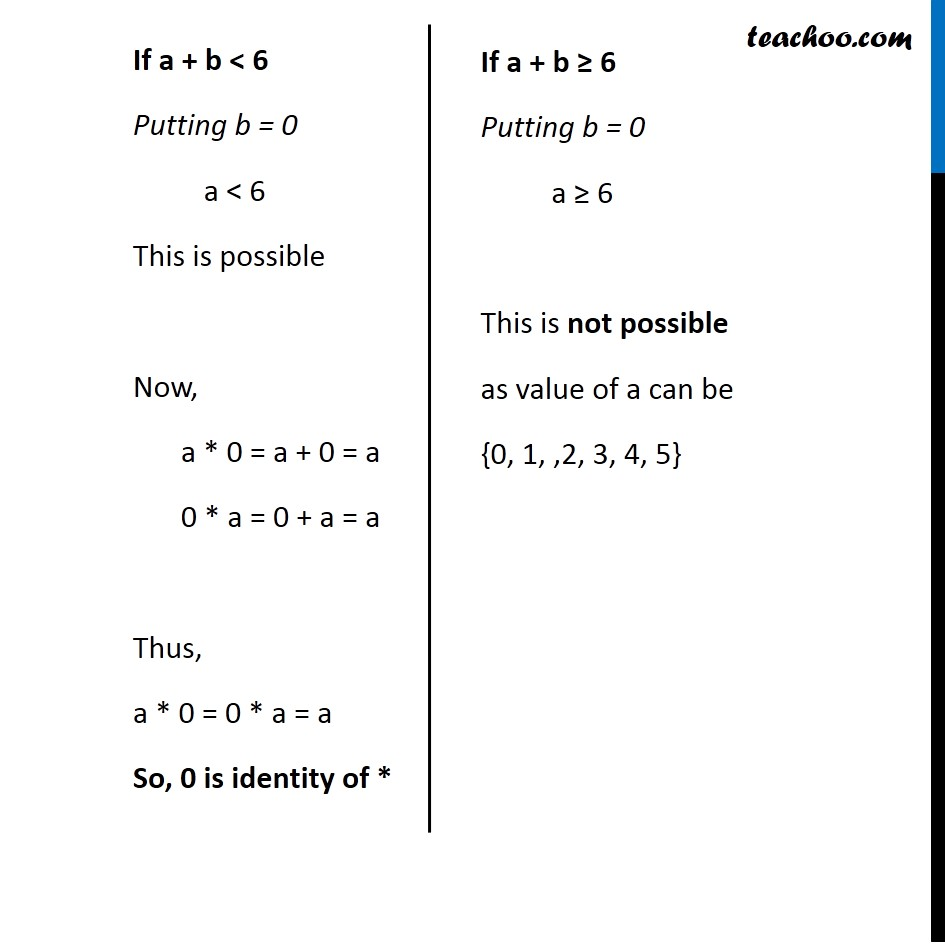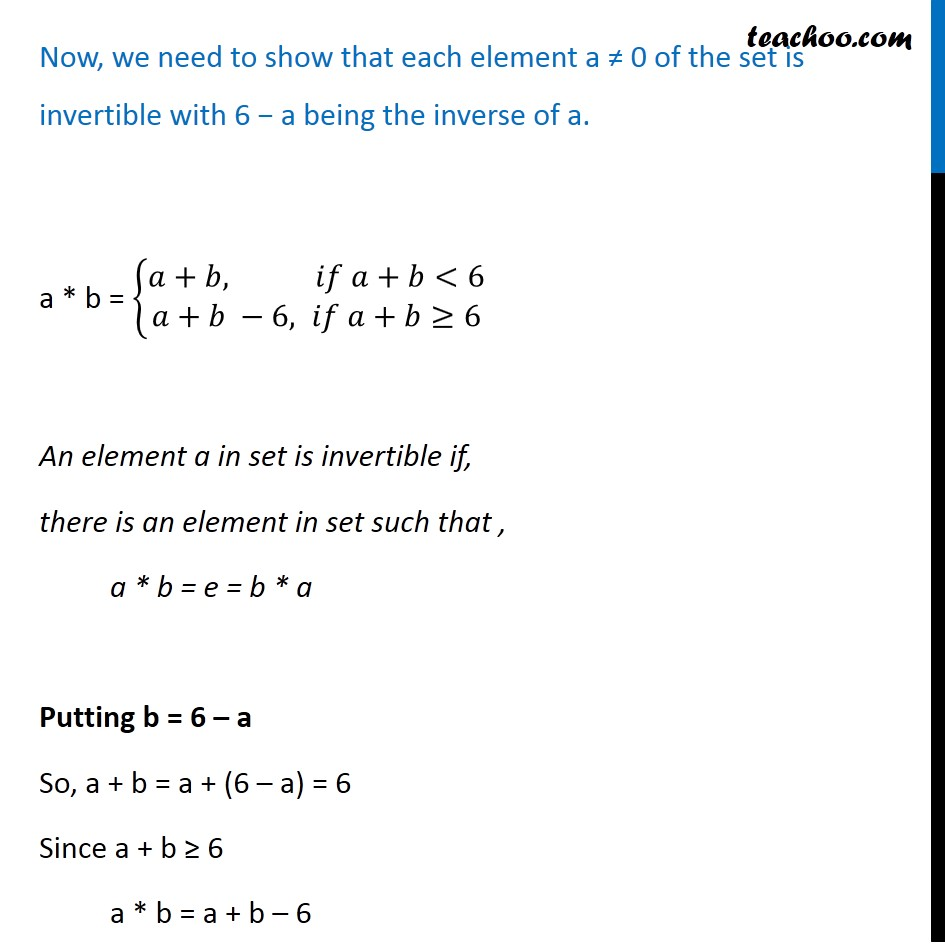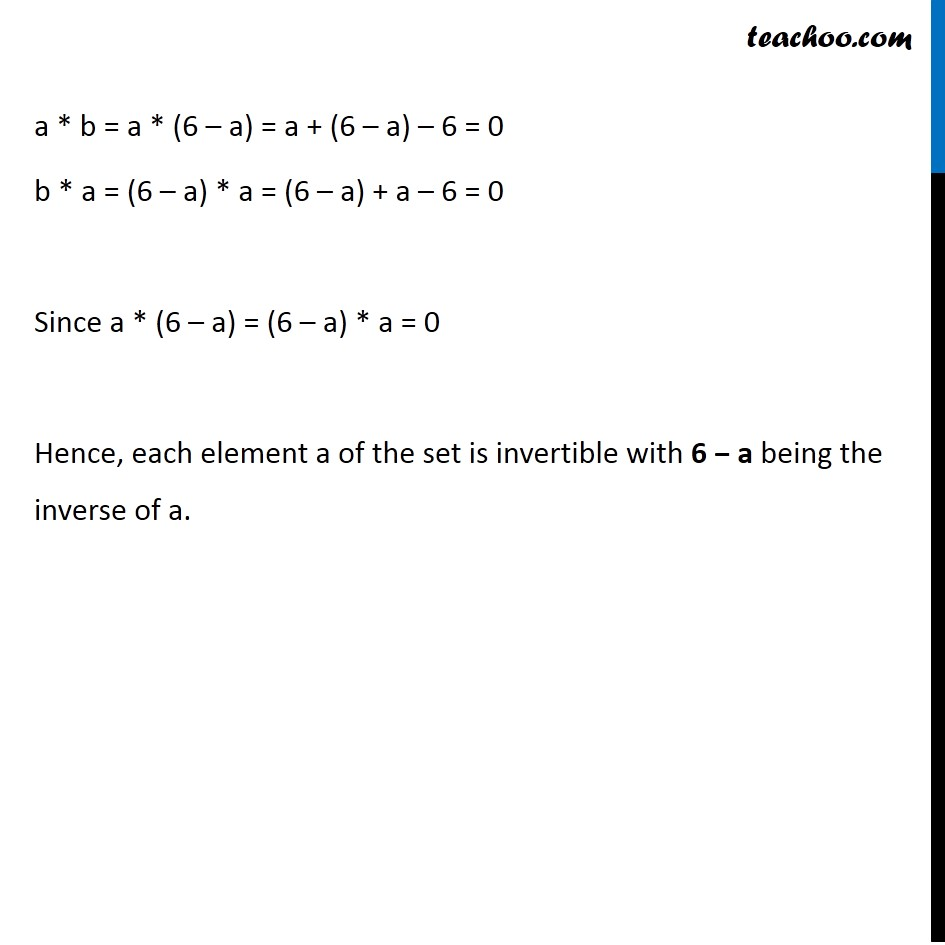Chapter 1 Class 12 Relation and Functions

Class 12
Important Questions for exams Class 12Introducing your new favourite teacher - Teachoo Black, at only ₹83 per month

### Transcript

Misc 14 Define a binary operation *on the set {0, 1, 2, 3, 4, 5} as a * b = {█(𝑎+𝑏, 𝑖𝑓 𝑎+𝑏<6@&𝑎+𝑏 −6, 𝑖𝑓 𝑎+𝑏≥6)┤ Show that zero is the identity for this operation and each element a ≠ 0 of the set is invertible with 6 − a being the inverse of a. e is the identity of * if a * e = e * a = a Checking if zero is identity for this operation If a + b < 6 Putting b = 0 a < 6 This is possible Now, a * 0 = a + 0 = a 0 * a = 0 + a = a Thus, a * 0 = 0 * a = a So, 0 is identity of * If a + b ≥ 6 Putting b = 0 a ≥ 6 This is not possible as value of a can be {0, 1, ,2, 3, 4, 5} Now, we need to show that each element a ≠ 0 of the set is invertible with 6 − a being the inverse of a. a * b = {█(𝑎+𝑏, 𝑖𝑓 𝑎+𝑏<6@&𝑎+𝑏 −6, 𝑖𝑓 𝑎+𝑏≥6)┤ An element a in set is invertible if, there is an element in set such that , a * b = e = b * a Putting b = 6 – a So, a + b = a + (6 – a) = 6 Since a + b ≥ 6 a * b = a + b – 6 a * b = a * (6 – a) = a + (6 – a) – 6 = 0 b * a = (6 – a) * a = (6 – a) + a – 6 = 0 Since a * (6 – a) = (6 – a) * a = 0 Hence, each element a of the set is invertible with 6 − a being the inverse of a. s